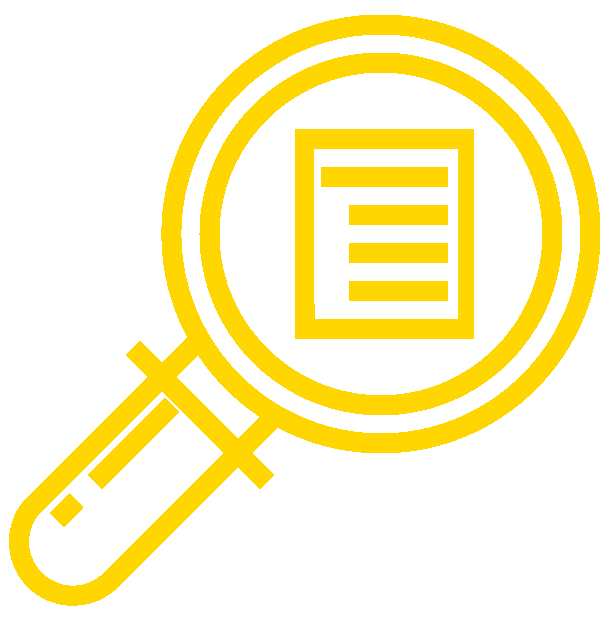## Learning OutcomesThe textbook content and assessments for Introduction to Statistics are aligned to the following learning outcomes.

## Module 1: Sampling and Data

• Recognize and differentiate between key terms.
• Apply various types of sampling methods to data collection.
• Create and interpret frequency tables.

## Module 2: Descriptive Statistics

• Display data graphically and interpret graphs: stemplots, histograms, and box plots.
• Recognize, describe, and calculate the measures of location of data: quartiles and percentiles.
• Recognize, describe, and calculate the measures of the center of data: mean, median, and mode.
• Recognize, describe, and calculate the measures of the spread of data: variance, standard deviation, and range.

## Module 3: Probability

• Understand and use the terminology of probability.
• Determine whether two events are mutually exclusive and whether two events are independent.
• Calculate probabilities using the Addition Rules and Multiplication Rules.
• Construct and interpret Contingency Tables.
• Construct and interpret Venn Diagrams.
• Construct and interpret Tree Diagrams.

## Module 4: Discrete Random Variables

• Recognize and understand discrete probability distribution functions, in general.
• Calculate and interpret expected values.
• Recognize the binomial probability distribution and apply it appropriately.
• Recognize the Poisson probability distribution and apply it appropriately.
• Recognize the geometric probability distribution and apply it appropriately.
• Recognize the hypergeometric probability distribution and apply it appropriately.
• Classify discrete word problems by their distributions.

## Module 5: Continuous Random Variables

• Recognize and understand continuous probability density functions in general.
• Recognize the uniform probability distribution and apply it appropriately.
• Recognize the exponential probability distribution and apply it appropriately.

## Module 6: Normal Distribution

• Recognize the normal probability distribution and apply it appropriately.
• Recognize the standard normal probability distribution and apply it appropriately.
• Compare normal probabilities by converting to the standard normal distribution.

## Module 7: The Central Limit Theorem

• Recognize central limit theorem problems.
• Classify continuous word problems by their distributions.
• Apply and interpret the central limit theorem for means.
• Apply and interpret the central limit theorem for sums.

## Module 8: Confidence Intervals

• Calculate and interpret confidence intervals for estimating a population mean and a population proportion.
• Interpret the Student’s t probability distribution as the sample size changes.
• Discriminate between problems applying the normal and the Student’s t distributions.
• Calculate the sample size required to estimate a population mean and a population proportion given a desired confidence level and margin of error.

## Module 9: Hypothesis Testing With One Sample

• Differentiate between Type I and Type II Errors
• Describe hypothesis testing in general and in practice
• Conduct and interpret hypothesis tests for a single population mean, population standard deviation known.
• Conduct and interpret hypothesis tests for a single population mean, population standard deviation unknown.
• Conduct and interpret hypothesis tests for a single population proportion.

## Module 10: Hypothesis Testing With Two Samples

• Classify hypothesis tests by type.
• Conduct and interpret hypothesis tests for two population means, population standard deviations known.
• Conduct and interpret hypothesis tests for two population means, population standard deviations unknown.
• Conduct and interpret hypothesis tests for two population proportions.
• Conduct and interpret hypothesis tests for matched or paired samples

## Module 11: The Chi Square Distribution

• Interpret the chi-square probability distribution as the sample size changes.
• Conduct and interpret chi-square goodness-of-fit hypothesis tests.
• Conduct and interpret chi-square test of independence hypothesis tests.
• Conduct and interpret chi-square homogeneity hypothesis tests.
• Conduct and interpret chi-square single variance hypothesis tests.

## Module 12: Linear Regression and Correlation

• Discuss basic ideas of linear regression and correlation.
• Create and analyze scatter plots.
• Create and interpret a line of best fit.
• Calculate and interpret the correlation coefficient.
• Use interpolation and extrapolation.
• Calculate and interpret outliers.

## Module 13: F-Distribution and the One-Way ANOVA

• Interpret the F probability distribution as the number of groups and the sample size change.
• Discuss two uses for the F distribution: one-way ANOVA and the test of two variances.
• Conduct and interpret one-way ANOVA.
• Conduct and interpret hypothesis tests of two variances.